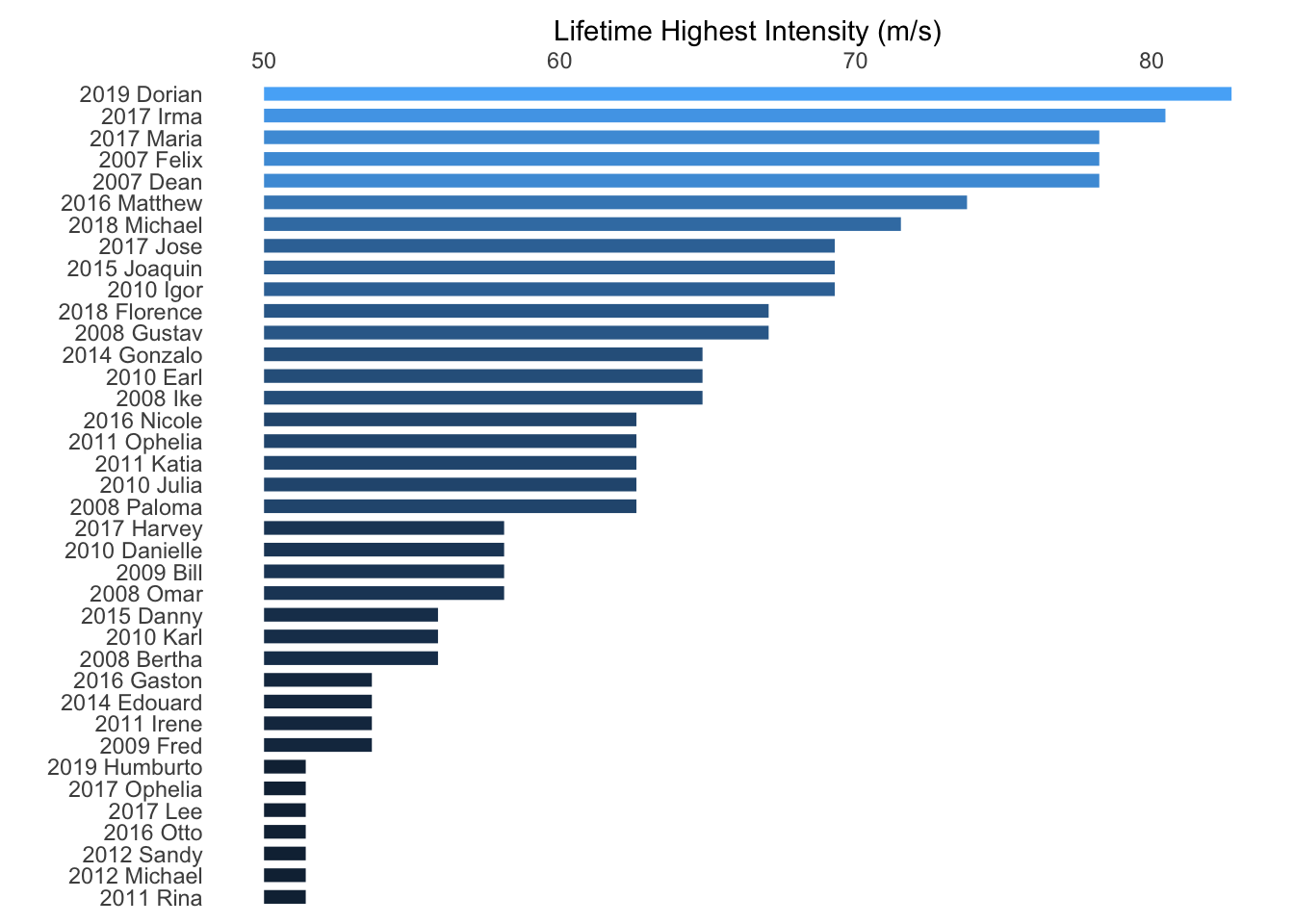Science gets validated through predictions. Using satellite derived wind speed estimates from tropical cyclones Elsner, Kossin, and Jagger (2008) showed that the strongest hurricanes got stronger in the period 1981-2006. In particular, they found that 15% (85th quantile) of all named storms across the North Atlantic had wind speeds exceeding 48.9 m/s (Table 1) with an upward trend of .63 m/s/yr in this quantile wind speed. They related this increase to a corresponding rise in ocean temperatures consistent with theory (Emanuel 1988). The oceans have continued to warm since that paper was published so we would predict that the upward trend in the intensity of the strongest hurricanes has continued.

To check this, consider all North Atlantic named storms (tropical storms and hurricanes) over the 13-year period 2007–2019 [data taken from Wikipedia]. NS and MH are the annual number of named storms and major hurricanes respectively.

Year <- c(2007, 2007, 2008, 2008, 2008, 2008, 2008, 2009, 2009, 2010, 2010, 2010, 2010, 2010,
2011, 2011, 2011, 2011, 2012, 2012, 2014, 2014, 2015, 2015, 2016, 2016, 2016, 2016,
2017, 2017, 2017, 2017, 2017, 2017, 2018, 2018, 2019, 2019)
Name <- c("Dean", "Felix", "Bertha", "Gustav", "Ike", "Omar", "Paloma", "Bill", "Fred",
"Danielle", "Earl", "Igor", "Julia", "Karl", "Irene", "Katia", "Ophelia", "Rina",
"Michael", "Sandy", "Edouard", "Gonzalo", "Danny", "Joaquin", "Gaston", "Matthew",
"Nicole", "Otto", "Harvey", "Irma", "Jose", "Lee", "Maria", "Ophelia", "Florence",
"Michael", "Dorian", "Humburto")
MPH <- c(175, 175, 125, 150, 145, 130, 140, 130, 120, 130, 145, 155, 140, 125, 120, 140, 140,
115, 115, 115, 120, 145, 125, 155, 120, 165, 140, 115, 130, 180, 155, 115, 175, 115,
150, 160, 185, 115)
LMI.df <- data.frame(Year, Name, MPH, MPS = MPH * .44704)

NS <- c(15, 16, 9, 19, 19, 19, 14, 8, 11, 15, 17, 15, 12)
MH <- c(2, 5, 2, 5, 4, 2, 0, 2, 2, 4, 6, 2, 2)
Year <- 2007:2019

Counts.df <- data.frame(Year, NS, MH)
# sum(MH)/sum(NS) * 100

We note that 20% of them had wind speeds exceeding 48.9 m/s. An increase of 5 percentage points.

suppressPackageStartupMessages(library(dplyr))
LMI.df %>%
arrange(desc(MPS))
##    Year     Name MPH     MPS
## 1  2019   Dorian 185 82.7024
## 2  2017     Irma 180 80.4672
## 3  2007     Dean 175 78.2320
## 4  2007    Felix 175 78.2320
## 5  2017    Maria 175 78.2320
## 6  2016  Matthew 165 73.7616
## 7  2018  Michael 160 71.5264
## 8  2010     Igor 155 69.2912
## 9  2015  Joaquin 155 69.2912
## 10 2017     Jose 155 69.2912
## 11 2008   Gustav 150 67.0560
## 12 2018 Florence 150 67.0560
## 13 2008      Ike 145 64.8208
## 14 2010     Earl 145 64.8208
## 15 2014  Gonzalo 145 64.8208
## 16 2008   Paloma 140 62.5856
## 17 2010    Julia 140 62.5856
## 18 2011    Katia 140 62.5856
## 19 2011  Ophelia 140 62.5856
## 20 2016   Nicole 140 62.5856
## 21 2008     Omar 130 58.1152
## 22 2009     Bill 130 58.1152
## 23 2010 Danielle 130 58.1152
## 24 2017   Harvey 130 58.1152
## 25 2008   Bertha 125 55.8800
## 26 2010     Karl 125 55.8800
## 27 2015    Danny 125 55.8800
## 28 2009     Fred 120 53.6448
## 29 2011    Irene 120 53.6448
## 30 2014  Edouard 120 53.6448
## 31 2016   Gaston 120 53.6448
## 32 2011     Rina 115 51.4096
## 33 2012  Michael 115 51.4096
## 34 2012    Sandy 115 51.4096
## 35 2016     Otto 115 51.4096
## 36 2017      Lee 115 51.4096
## 37 2017  Ophelia 115 51.4096
## 38 2019 Humburto 115 51.4096

Plot as an ordered bar plot.

LMI.df <- LMI.df %>%
mutate(NAME = paste(Year, Name))

suppressPackageStartupMessages(library(ggplot2))
suppressPackageStartupMessages(library(forcats))

ggplot(data = LMI.df) +
geom_segment(aes(x = reorder(NAME, MPS), y = 50,
xend = reorder(NAME, MPS), yend = MPS,
color = MPS), size = 2.5) +
coord_flip() +
scale_y_continuous(position = 'right') +
xlab("") + ylab("Lifetime Highest Intensity (m/s)") +
theme_minimal() +
theme(panel.grid.major = element_blank(),
panel.grid.minor = element_blank(),
legend.position = "none")percentile <- (cumsum(table(LMI.df$MPS)) + (sum(NS) - sum(MH)))/sum(NS) percentile.df <- as.data.frame(percentile) percentile.df$MPS <- as.numeric(rownames(percentile.df))

p85ws <- round(approx(x = percentile.df$percentile, y = percentile.df$MPS, xout = .85)$y, 1) Further we note that the 85th quantile wind speed has increased from 48.9 m/s to 52.9 m/s which is an increase of 4 m/s and which matches an extrapolation by taking the trend of .63 m/s/yr from Table 1 of Elsner et al. (2008) and multipling it by 7 (mid point number of years in a 13-year period). [48.9 + .63 * 7 = 53.3 m/s]. p95 <- round(approx(x = percentile.df$MPS, y = percentile.df$percentile, xout = 60.3)$y, 1)
p95ws <- round(approx(x = percentile.df$percentile, y = percentile.df$MPS, xout = .95)\$y, 1)

Furthermore we note that 5% of the storms exceeded 60.3 m/s over the earlier period (Table 1, 95th quantile) but has increased to 10% over the later period. The 95th quantile wind speed has increased from 60.3 m/s to 67.5 m/s consistent with extrapolating the trend [60.3 + .81 * 7 = 66 m/s].

It is hard to argue against this straightforward post publication analysis and the results raise the question of why this work was largely ignored when writing “state-of-the-knowledge” reports on hurricanes and climate change.